# One-Frame Views of Flat-Space Dynamics

Constant Proper-Acceleration: The Movie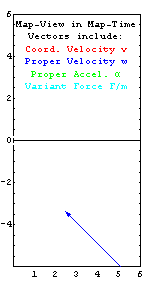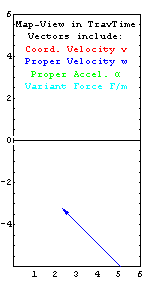A few things to note:
• Axes represent position measured by a system of co-moving...
...yardsticks and synchronized clocks we call "the map".
• Frame-dependent simultaneity is thus here defined by map clocks.
• Map-Time t (elapsing on the left) moves slower than...
... Traveler Time tau (elapsing on the right) especially at high speeds.
• Coordinate-Velocity (v=dx/dt) has an upper limit.
• Proper-Velocity (w=dx/dtau) doesn't.
• The magnitude of Force per unit Mass F/m is nearly...
...as large as that of Proper-Acceleration alpha, except...
...when velocity is both large and perpendicular to acceleration.
• Force parallels Acceleration when velocity is small or perpendicular.
• At higher speeds, Force becomes parallel to the Velocity line.
• Einstein assures us that these flat-space dynamics are locally useful...
...even in curved spacetime, if we invoke affine-connection forces.
• All quantities are in dimensionless units e.g. [ly], [y], [ly/y^2]...
...except velocity arrows, which are scaled back by 0.6 to fit.
• Puzzler: If the distance units on your monitor are [ly],...
how many [y] are there per display second?

For a more static look at constant (unit rightward) proper-acceleration, check out this multiple-exposure of the trajectory in the movie above (left panel), taken at equal traveler time intervals. The comments above also apply there. On the right, find an analogous multiple-exposure snapshot from a movie involving constant (unit rightward) frame-variant force. Coming soon to screens in your neighborhood...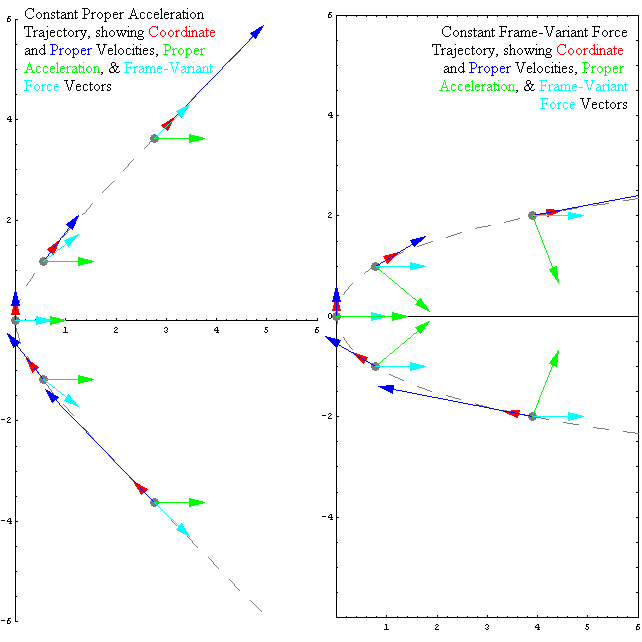The "component color" plots below offer a more comprehensive view of the relationship TO coordinate-velocity OF: proper-velocity (upper left), frame-variant force (upper right), coordinate-acceleration (lower left), and proper-acceleration (lower right). These plots connect vector positions in coordinate-velocity space, with a plotted vector variable whose log[magnitude] and direction are denoted by brightness/saturation and cyclic hue, respectively.

The simplest plot is on the upper left. Proper-velocity v in all cases points radially outward, in the same direction as the coordinate-velocity vector from the origin to any given point on the plot. In that sense, this upper-left plot can also be used as key for associating colors with directions. For example, red is up, cyan is down, chartreuse is rightward, indigo is to the left, etc. The outer edge of the circle corresponds to a coordinate-velocity of v=c. The proper-velocity magnitude (brightness) increases monotonically from the center to the annular unit-value-intensity algorithm-change ring when proper-velocity (w) reaches lightspeed c at a radius of v=1/Sqrt=.707c. Beyond that proper-velocity's increasing magnitude is denoted by increasing color saturation. Proper-velocity eventually becomes infinitely large (hence white), but only for values of coordinate-velocity which are extremely close to c (the circle's edge).

The remaining panels plot the direction and magnitude of a force (upper right panel) or acceleration (lower panel) vector, relative to a chosen reference value (generally rightward pointing of unit length) for an associated acceleration or force. For example, from the panels on the right one can see that, for most of coordinate-velocity space, proper-acceleration (alpha) and force-per-unit-mass (F/m) have essentially the same yellow-green (rightward) hue and brightness (unit-magnitude) i.e. F ~ m*alpha. This is not true for coordinate acceleration plotted in the lower left panel, which falls to zero for the given unit force whenever an object approaches the speed of light. It's also easy to see on the upper right that when F/m deviates from alpha, it's value is always less than alpha's, and that this deviation is only severe when coordinate velocity is large and perpendicular to acceleration. As we will show in the section to follow, constant proper-acceleration trajectories correspond to horizontal lines across this circle. When velocity and acceleration are unidirectional, F = m*alpha exactly regardless of speed.

The lower right plot shows the converse, i.e. when alpha does deviate from F/m at high speeds, it's value (i.e. brightness) is always greater than the fixed value (1 [ly/y^2]) for F/m. This increase in acceleration magnitude (hence color saturation or whiteness) for the given rightward force peaks when the object's velocity is both large, and perpendicular to the force direction. This effect is directly responsible for the magnetism associated with a line of moving charges, and hence for the workability of electric motors, microphones, and even refrigerator magnets. Also note that the deviation between force and acceleration directions is greatest when the accelerated object's velocity is large and near (but not exactly on) the force direction. All fixed force trajectories end up in this region (see below), except those for which the initial velocity transverse to the force is zero (and hence F = m*alpha exactly).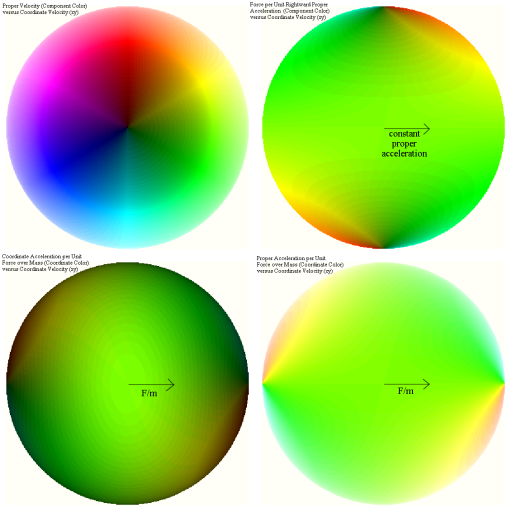A more quantitative comparison of coordinate-velocity, proper-velocity, proper-acceleration and frame-variant force directions is possible if we plot constant proper-acceleration and constant-force trajectories, e.g. across the coordinate-velocity circles shown above.

Two cases are treated below:
• (left) motion with constant proper-acceleration (and hence a constant value of coordinate-velocity perpendicular to the acceleration), and
• (right) motion with a constant force from the map-frame's perspective (and hence a constant value of proper-velocity transverse to the fixed force direction).
The center circle in each plot corresponds to the coordinate-velocity circle in the component-color plots above. Sample vector sets have been drawn in schematically to provide clues on use of these plots (coordinate-velocity is red, proper-velocity is blue, proper-acceleration is green, and F/m is cyan).

Basically as one slides the velocity arrowheads along a given set of appropriately colored lines, the remaining non-horizontal arrowhead moves so that the force is parallel to acceleration at low speeds, but parallel to velocity at high speeds. Note that for constant proper-acceleration (left panel) the velocity and force directions seem to uniformly approach a common limiting (high speed) value from opposite directions. Also note that for constant force motion (right panel) the magnitude of proper-acceleration remains constant, but that its direction changes. Hence a space-ship capable of constant proper-acceleration could likely "fake" constant force motion with respect to a given map-frame simply by changing the direction of it's thrusters as a function of time.

Lastly, note from the plot on the right that the proper-acceleration due to a given map-frame force on it increases as it's motion perpendicular to that force increases. Thus the effect of the attraction (i.e. the felt acceleration) of a fixed charge to a fixed line of charges may be less than that seen when the charge is moving parallel to the fixed line. A bit of thought allows one to connect this observation of dynamics in spacetime, quantitatively, to the origins of magnetism (i.e. the Lorentz and Biot-Savart Laws) given electrostatics alone. On the subject of dynamics, also look for more on the integrals of constant force (perhaps even another movie) in the days ahead...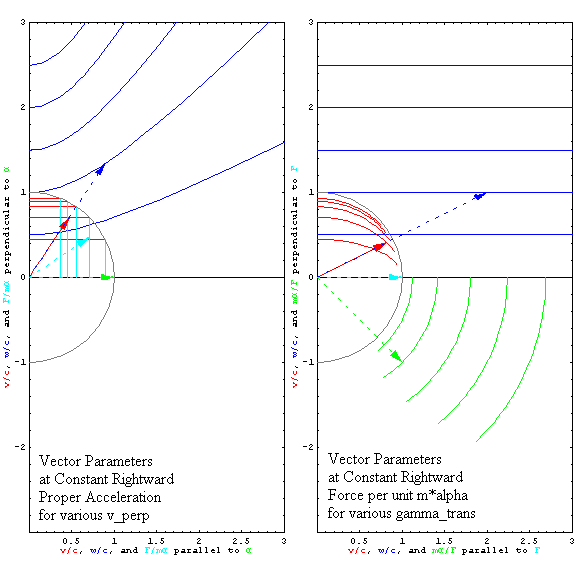This page is http://www.umsl.edu/~fraundor/anyspeed/conaccel.html. Although there are many contributors, the person responsible for errors is P. Fraundorf. This site is hosted by the Department of Physics and Astronomy (and Center for Molecular Electronics) at UM-StL. Mindquilts site page requests ~2000/day approaching a million per year. Requests for a "stat-counter linked subset of pages" since 4/7/2005: .